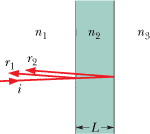# Interference in Thin Films, figuring out the phases

• emmy
In summary, the conversation discusses a problem involving interference of light waves in a thin layer of material and determining the phase interference to be either maximum or minimum. The given parameters for the problem are also provided. The individual is seeking clarification on why the equation for a maximum involves multiplying the integer m by 2 and adding 1/2. The conversation also references an online resource with helpful animations and suggests drawing a wave to better understand the concept.

## Homework Statement

I don't have a question on a specific problem, I am more caught up in the determination of the phases- I'll give a general problem to use as an example:

In Fig. 35-41, light is incident perpendicularly on a thin layer of material 2 that lies between (thicker) materials 1 and 3. The waves of rays r1 and r2 interfere, and here we consider the type of interference to be either maximum (max) or minimum (min). We are given:

n1=1.32
n2=1.75
n3=1.39
we want to find a max
for the 3rd least thickness of L (in nm)
λ=382nm (in air)

Figure:rays are tilted for clarity

## Homework Equations

Since we want a maximum, the general equation for a maximum would be:
2L=(m+1/2)λ/n2

## The Attempt at a Solution

in the case of this problem, we add an additional λ/2 for the incidence of r1 on n2.
so my real question is this-

why is it that you multiply m by 2 to end up with 2m+1 in this case? when do you just use (m+1)λ vs (2m+1/2)λ

I've been reading and researching for hours now, so any help would truly be appreciated.

High-speed to slow speed you get a phase inversion.
http://www.kettering.edu/physics/drussell/Demos/reflect/reflect.html [Broken]
... it has neat animations of the effect for string, you have to scroll down for the general.

That 2m+1 gives you an odd number - do you see why an odd number is needed?

The trick is to try drawing a wave as it propagates through the film. What happens if it's an even number?

Last edited by a moderator: